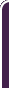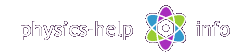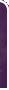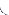special relativity, rest mass, Lorentz transformation, relativistic effects, time dilation, proper time, length contraction, proper length, simultaneity violation, relativistic mass, relativistic momentum, relativistic force, rest energyhomephysics online guidephysics help my info terms of use contactsWhat is Physics? Mechanics Electricity and Magnetism Electric Field Gauss' Law about below subjects Electric Potential Capacity Direct Current Magnetic Field Magnetic Field Laws Magnetic Interactions Electromagnetic Induction Maxwell's Equations Oscillations and Waves Simple Harmonic Motion Damped Harmonic Motion Driven Harmonic Motion Electric Oscillation Alternating Current Wave Motion Elastic Waves Electromagnetic Waves Optics Light Waves Geometrical Optics Interference Polarization Diffraction Fraunhofer Diffraction Dispersion, Absorption, Diffusion Doppler Effect Thermodynamics Ideal Gas Molecular Statistics Transport Phenomena First Law of Thermodynamics Second and Third Laws of Thermodynamics Imperfect Gas Liquids Solids Quantum Physics Thermal Radiation Quantum Properties of Light Wave Properties of Particles Planetary Model of Atom X-Rays Particle in Potential Well Pauli Exclusion Principle Nuclear Physics Solid State Physics Appendices# Special Relativity

SI units & Physics constants

Special relativity investigates motion of objects at high speeds approaching the speed of light in inertial reference framesHere (all units see here):

x, y, z is fixed inertial reference frame

x', y', z' is inertial reference frame moving at constant velocity along x-axis

v is velocity of frame x', y', z' with respect to frame x, y, z

mo is rest mass of the object measured in frame x', y', z'

t is instant of time of position of the object measured in frame x, y, z

t' is proper instant of time of position of the object measured in frame x', y', z'is proper force with components Fox, and Foy acting on the object measured in frame x', y', z'

c is speed of light

## General formulas

Lorentz transformation between coordinates and time for frames x', y', z' and x, y, zInverse transformation## Einstein's Postulates of Special Relativity

Relativity Postulate. All laws of physic are the same (have the same form) in every inertial reference frame

Speed of Light Postulate. The speed of light in vacuum, measured in any inertial reference frame, always has the same value, no matter how the source of light and the object are moving relative to each other

## Relativistic effects

Time Dilation. The time interval between two events, measured by observer standing in fixed frame x, y, zwhereis proper time interval between the same two events taking place at the same point, measured by observer standing in moving frame x', y', z'

Length Contraction. The length or distance between two points in direction of motion, measured at the same time by observer standing in frame x, y, zwhere Lo is proper length or distance between the same points, measured by observer standing in frame x', y', z'

Simultaneity Violation. The time interval between two events which are simultaneous in frame x', y', z', measured by observer standing in frame x, y, zwhere Lo is proper distance between the points where the simultaneous events take place, measured by observer standing in frame x', y', z'

Relativistic law of adding velocities. The velocity of object, measured by observer standing in frame x, y, zwhere uo is relative velocity of the object moving  in x-direction, measured by observer standing in frame x', y', z'

Relativistic mass. The mass of object, measured by observer standing in frame x, y, zwhere mo is rest mass, measured by observer standing in frame x', y', z'

Relativistic momentum. The linear momentum of object moving with velocityRelativistic force vector. The x and y components of forceacting on object, measured by observer standing in frame x, y, zwhere Fox and Foy are components of proper force, measured by observer standing in frame x', y', z'

The relativistic Newton's Second Law for motionTotal energy of mass moving with speed vwhere mo is rest mass

Rest energy of massRelativistic kinetic energy of object moving with speed vRelation between relativistic energy and momentumhome  |  physics online guide  |  physics help  |  my info  |  terms of use  |  contacts Dr. Nikitin © 2010